In my last post I used structReduce. I thought I’d post some more examples of what you can use reducers for.

The classic example is to sum the values in an array.

```data = [1,2,3,4,5,6,7,8,9,10];

result = data.reduce(function(accumulator, element) {
accumulator += element;
return accumulator;
}, 0);

writeDump(result); // 55
```

A more interesting example is to take a typical array of objects and convert it to a struct using the id as the key. key lookups are fast so you may want to do something like this when you don’t want to have to keep searching the array.

```data = [
{"id":100, label:"One Hundred"},
{"id":200, label:"Two Hundred"},
{"id":300, label:"Three Hundred"}
];

result = data.reduce(function(accumulator, element) {
accumulator[element.id] = element.label;
return accumulator;
}, {});
```

The result is a struct: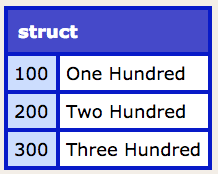An another example is taking something like a query string and converting it to a struct:

```input = "a=1&b=2&c=3";
data = input.listToArray("&");

result = data.reduce(function(accumulator, element) {
var key = listFirst(element, "=");
var value = listLast(element, "=");
accumulator[key] = value;
return accumulator;
}, {});
```

The result is: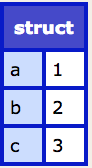```data = [
10, [20, 21, 22], 30, [40, 42, 43]
];

result = data.reduce(function(accumulator, element) {
accumulator.append(element, isArray(element));
return accumulator;
}, []);
```

The result is: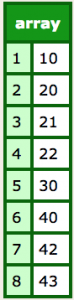So far these examples have all been based on an array (arrayReduce), but you can also use a reducer on structs (structReduce). For example, you can also use it to group things. In this example, I want to group capital cities by population in millions (rounded).

```populations = {
"Tokyo": 13.4,
"Beijing": 20.7,
"Delhi": 16.8,
"Manila": 12.9,
"Moscow": 11.5
};

// grouping by population
groupByPopulation = populations.reduce(function(accumulator, key, value) {
var groupKey = round(value);
if (!accumulator.keyExists(groupKey)) {
accumulator[groupKey] = [];
}
accumulator[groupKey].append(key);
return accumulator;
}, {});
```

The output has a key for each ‘million’ banding and the countries in that meet that criteria.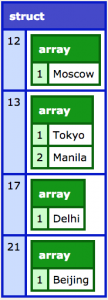You can even use reduce on query objects:

```data = QueryNew("id,label,active",
"integer,varchar,bit",
[
{"id":100, label:"One Hundred", active: 1},
{"id":200, label:"Two Hundred", active: 0},
{"id":300, label:"Three Hundred", active: 1}
]
);

result = data.reduce(function(accumulator, element) {
accumulator[element.id] = {
label: element.label,
active: element.active ? true : false
};
return accumulator;
}, {});

writeDump(data);
writeDump(result);
```

This will output (I’ve dumped out the original query as well just to make it clear).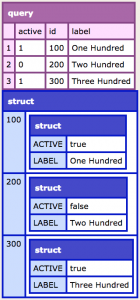Hopefully this post has given you some ideas of where reduce may be useful in your code.